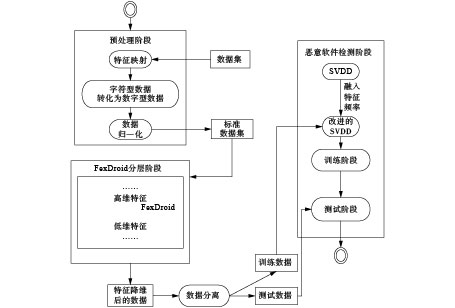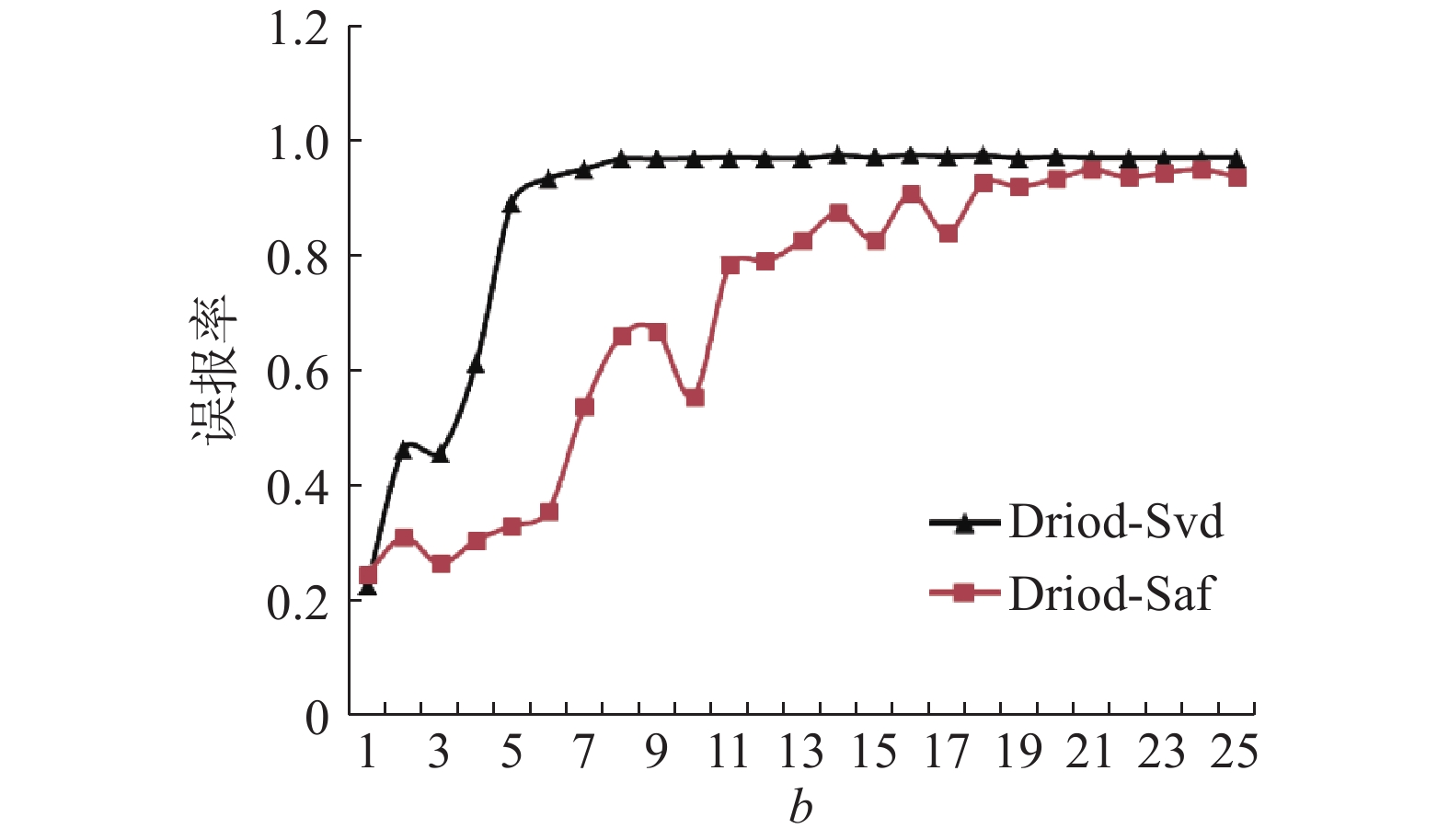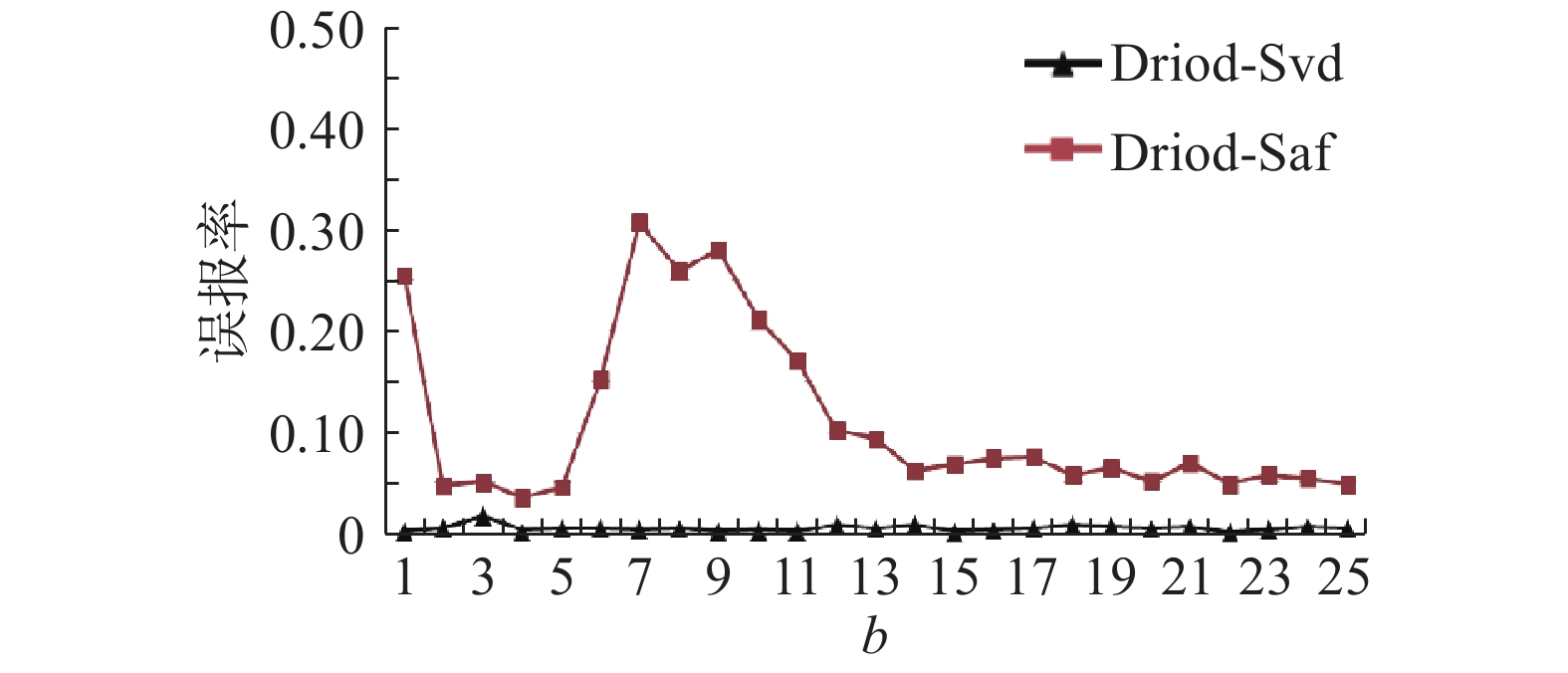﻿ 依特征频率的安卓恶意软件异常检测的研究
«上一篇文章快速检索 高级检索

 智能系统学报2018, Vol. 13Issue (2): 168-173  DOI: 10.11992/tis.2016090160

### 引用本文ZHANG Yuling, YIN Chuanhuan. Android malware outlier detection based on feature frequency[J]. CAAI Transactions on Intelligent Systems, 2018, 13(2): 168-173. DOI: 10.11992/tis.201609016.### 文章历史

Android malware outlier detection based on feature frequency
ZHANG Yuling, YIN ChuanhuanSchool of Computer and Information Technology, Beijing Jiaotong University, Beijing 100044, China
Abstract: Due to the advantages of open source and portability, Android has become a mobile OS with the largest market share. Various attacks toward Android also emerge in endlessly, the Android-oriented detection for malwares has become a quite important link recently in the field of mobile safety. The problems to be faced include difficult collection of malicious software, imbalanced proportion of the abnormal samples and normal samples. In order to effectively overcome the above difficulties, Droid-Saf framework was proposed, a data processing scheme revealing the implicit characteristics of data was proposed in the framework; the hidden information contained in the sample was treated as a new feature; in modeling, the sample features were integrated into the algorithm and dynamic slack variables were established. Static analytic method was applied to decompile apk, the improved svdd single classifier was used for classification, the deficiency of difficult collection of abnormal software in the system for detecting malicious software was overcome, the rate of missing report and the misjudgment rate of abnormal detection were lowered. The Experimental results verified the effectiveness and applicability of the algorithm.
Key words: Android system    malware    data mining    abnormal detection    svdd    implicit characteristics    single classifier    feature frequency

1 静态分析与动态分析

2 异常检测

3 SVDD的基本原理及改进方案

SVDD首先通过核函数将输入空间映射到一个高维空间，在这个高维空间构造一个包含所有训练样本点的球体；在球面上的样本点即为SVDD所求得的支持向量。假设模型f(x; w)表示一类紧密的有界数据集，SVDD的优化目标就是求一个中心为a半径为R的最小球面，而且使训练集X的所有样本都落在此球体内。它的原理和SVM很像，类比于SVM，定义一个最小化问题：

 $F(R,{{a}},\xi ) = {R^2} + C\sum\limits_i {{\xi _i}}$ (1)

 $\begin{array}{l}{\left( {{{x}_{{i}}} - {{a}}} \right)^2} \leqslant {R^2} + {\xi _i}\\{{s}}{{.t}}{{.}}\;\;\;\;{\xi _i} \geqslant 0,\;\;\;\;\forall i\end{array}$ (2)

 $\begin{array}{l}L = \sum\limits_i {{\alpha _i}({{{x}}_i} \cdot {{{x}}_i}) + \sum\limits_{i,j} {{\alpha _i}{\alpha _j}({{{x}}_i} \cdot {{{x}}_j})} } \\{{s.t.}}\;\;\;\;\;\sum\limits_i {{\alpha _i} = 1,\;\;\;\;0 \leqslant {\alpha _i} \leqslant C} \end{array}$ (3)

 ${({{z}} - {{a}})^{{T}}}({{z}} - {{a}}) \leqslant {R^2}$ (4)

 $({{z}} \cdot {{z}}) - 2\sum\limits_i {{\alpha _i}({{z}} \cdot {{{x}}_i}) + \sum\limits_{i,j} {{\alpha _i}{\alpha _j}({{{x}}_i} \cdot {{{x}}_j}) \leqslant {R^2}} }$ (5)

 ${{K}}({{z}} \cdot {{z}}) - 2\sum\limits_i {{\alpha _i}{{K}}({{z}} \cdot {{{x}}_i}) + \sum\limits_{i,j} {{\alpha _i}{\alpha _j}K({{{x}}_i} \cdot {{{x}}_j}) \leqslant {R^2}} }$ (6)

 $\begin{array}{l}{c_i} = \displaystyle\frac{{\sum\limits_{j = 1}^m {{P_{ij}}} }}{{\max (\sum\limits_{j = 1}^m {{P_{ij}}} )}}\\{{s.t.}}\;\;\;i = 1,2, \cdots ,n\end{array}$ (7)

 $\begin{array}{c}\min \;\;\;F(R,{{a}},\xi ) = {R^2} + \sum\limits_{i = 1}^n {{c_i}{\xi _i}} \\{{s.t.}}\;\;\;{\left\| {{{{x}}_i} - {{a}}} \right\|^2} \leqslant {R^2} + {\xi _i}\\{\xi _i} \geqslant 0,i = 1,2, \cdots ,n\end{array}$ (8)

 $\begin{array}{c}L({{a}},R,\xi ,\alpha ,\gamma ) = {R^2} + \sum\limits_{i = 1}^n {{c_i}{\xi _i} - } \sum\limits_{i = 1}^n {{a_i}[{R^2} + {\xi _i}} - \\({{{x}}_i} \cdot {{{x}}_i}) + 2({{a}} \cdot {{{x}}_i}) - ({{a}} \cdot {{a}})] - \sum\limits_{i = 1}^n {{\gamma _i}{\xi _i}} \end{array}$ (9)

 $\begin{array}{c}\sum\limits_i {{\alpha _i} = 1} \\0 \leqslant {\alpha _i} \leqslant {c_i}\\{{s.t.}}\;\;\;i = 1,2, \cdots ,n\end{array}$ (10)

4 实验部分 4.1 实验流程

4.2 数据提取

4.3 评价指标

FP：将样本判定为正常样本，实际为恶意样本的数量。

TN：将样本判定为恶意样本，实际为恶意样本的数量。

TP：将样本判定为正常样本，实际为正常样本的数量。Download: 图 1 异常检测流程框架 Fig. 1 The framework of anomaly detection process表 1 实验评价指标 Tab.1 The evaluating indicator of experiment

FN：将样本判定为恶意样本，实际为正常样本的数量。

4.4 实验步骤Download: 图 2 RBF核函数的实验对比 Fig. 2 The experimental comparison of RBF kernel

Droid-Saf对恶意软件检测的正确率基本达到100%，参数c对结果并没有影响，因此对参数不敏感；但是Droid-Svd的部分漏报率大于0，c越大，误判率越高，漏报率也越高。为了详细说明细节，表2列出了Droid-Svd的实验情况。

c=0.05时，参数b对基于多项式核函数的Droid-Saf并没有作用，漏报率基本为0，误报率明显低很多。表 2 基于多项式核函数的Droid-Svd实验的平均值 Tab.2 The experimental FPR comparison of Polynomial kernel functionDownload: 图 3 多项式核函数的实验对比 Fig. 3 The experimental comparison of Polynomial kernel function
4.5 实验总结表 3 实验总结的平均结果 Tab.3 The summarized average results of the experiments

5 结束语

  微头条. Gartner: 2016全球手机出货预计19.59亿部[EB/OL]. http://www.wtoutiao.com/p/19cnOtt.html. (0)  中文业界资讯站. 2015年Android恶意软件样本数量超230万[EB/OL]. [2017-05-13]. http://www.cnbeta.com/articles/478843.html. (0)  杨威, 肖旭生, 李邓锋, 等. 移动应用安全解析学: 成果与挑战[J]. 信息安全学报, 2016, 1(2): 1-14. YANG Wei, XIAO Xusheng, LI Dengfeng, et al. Security analytics for mobile apps: achievements and challenges[J]. Journal of cyber security, 2016, 1(2): 1-14. DOI:10.11959/j.issn.2096-109x.2016.00031 (0)  AVDIIENKO V, KUZNETSOV K, GORLA A, et al. Mining apps for abnormal usage of sensitive data[C]//Proceedings of 37th IEEE International Conference on Software Engineering. Florence, Italy, 2015: 426–436. (0)  JUSZCZAK P. Learning to recognise: a study on one-class classification and active learning[D]. TU Delft, the Netherlands: Delft University of Technology, 2006. (0)  ZHOU W, ZHOU Y, GRACE M, et al. Fast, scalable detection of piggybacked mobile applications[C]//Proceedings of the third ACM conference on Data and application security and privacy. [s.l.], ACM, 2013: 185–196. (0)  TAX D M J, DUIN R P W. Support vector data description[J]. Machine learning, 2004, 54(1): 45-66. DOI:10.1023/B:MACH.0000008084.60811.49 (0)  ZHOU Wu, ZHOU Yajin, GRACE M, et al. Fast, scalable detection of " piggybacked” mobile applications[C]//Proceedings of the Third ACM Conference on Data and Application Security and Privacy. San Antonio, Texas, USA, 2013: 185–196. (0)  GRACE M, ZHOU Yajin, ZHANG Qiang, et al. Riskranker: scalable and accurate zero-day Android malware detection[C]//Proceedings of the 10th International Conference on Mobile Systems, Applications, and Services (MOBISYS). Lake District, UK, 2012: 281–294. (0)  WU Songyang, WANG Pan, LI Xun, et al. Effective detection of android malware based on the usage of data flow APIs and machine learning[J]. Information and software technology, 2016, 75: 17-25. DOI:10.1016/j.infsof.2016.03.004 (0)  YUAN Zhenlong, LU Yongqiang, WANG Zhaoguo, et al. Droid-Sec: deep learning in android malware detection[C]//Proceedings of the 2014 ACM Conference on SIGCOMM. Chicago, Illinois, USA, 2014: 371–372. (0)  SHEEN S, ANITHA R, NATARAJAN V. Android based malware detection using a multifeature collaborative decision fusion approach[J]. Neurocomputing, 2015, 151: 905-912. DOI:10.1016/j.neucom.2014.10.004 (0)  TAM K, KHAN S J, FATTORI A, et al. CopperDroid: automatic reconstruction of android malware behaviors[OL/EB]/. [2016-03-24]. https://www.researchgate.net/publication/300925104. (0)  BURGUERA L, ZURUTUZA U, NADJM-TEHRANI S. Crowdroid: behavior-based malware detection system for android[C]//Proceedings of the 1st ACM Workshop on Security and Privacy in Smartphones and Mobile Devices. Chicago, Illinois, USA, 2011: 15–26. (0)  TAM K, KHAN S J, FATTORI A, et al. CopperDroid: Automatic Reconstruction of Android Malware Behaviors[C]//Proceedings of Annual Network and Distributed System Security (NDSS). San Diego, United States, 2015. (0)  FARUKI P, BHANDARI S, LAXMI V, et al. DroidAnalyst: synergic app framework for static and dynamic app analysis[M]//ABIELMONA R, FALCON R, ZINCIR-HEYWOOD N, et al. Recent Advances in Computational Intelligence in Defense and Security. Cham: Springer, 2016: 519–552. (0)  TAX M J D, DUIN ROBERT P W. Support vector domain description[J]. Pattern recognition letters, 1999, 20(11/12/13): 1191-1199. (0)  HASTIE T, TIBSHIRANI R, FRIEDMAN J. Unsupervised learning[M]//HASTIE T, TIBSHIRANI R, FRIEDMAN J. The Elements of Statistical Learning. New York, USA: Springer, 2009: 485–585. (0)  CRISTIANINI N, SHAWE-TAYLOR J. 支持向量机导论[M]. 李国正,译. 北京: 电子工业出版社, 2004: 57–61. CRISTIANINI N, SHAWE-TAYLOR J. An introduction to support vector machines and other kernel-based learning methods[M]. LI Guozheng, Trans. Beijing: Publishing House of Electronics Industry, 2004: 57–61. (0)  罗隽, 丁力, 潘志松, 等. 异常检测中频率敏感的单分类算法研究[J]. 计算机研究与发展, 2007, 44(Z2): 235-239. LUO Jun, DING Li, PAN Zhisong, et al. Research on sequence-call-frequency-based one-class algorithm in abnormal detection[J]. Journal of computer research and development, 2007, 44(Z2): 235-239. (0)  张玉玲, 尹传环. 基于SVM的安卓恶意软件检测[J]. 山东大学学报: 工学版, 2017, 47(1): 42-47. ZHANG Yuling, YIN Chuanhuan. Android malware detection based on SVM[J]. Journal of Shandong university: engineering science, 2017, 47(1): 42-47. (0)